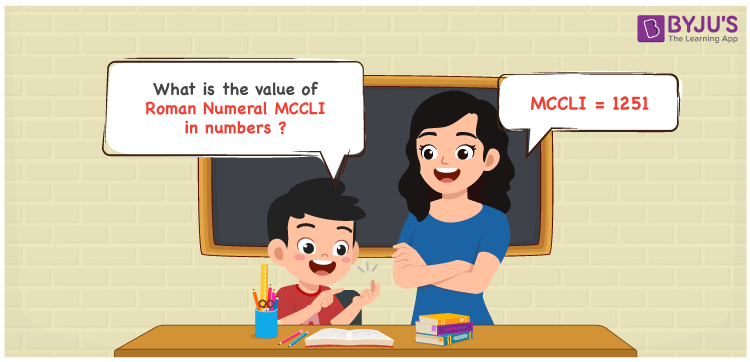Checkout JEE MAINS 2022 Question Paper Analysis : Checkout JEE MAINS 2022 Question Paper Analysis :

# MCCLI Roman Numerals

MCCLI Roman Numerals is 1251. This article provides a wide knowledge of transforming MCCLI to numbers in a simple to understand way. Get a good grip on the basic concepts by revising it on a daily basis and face your exams more confidently. These roman numerals conversion articles have the details required to understand the conversion effortlessly. Therefore, MCCLI can be indicated as 1251 in numbers.

 Number Roman Numeral 1251 MCCLI## How to Write MCCLI Roman Numerals in Numbers?

Students will learn the process used to convert MCCLI to numeric form by reading the steps here.

MCCLI = M + C + C + L + 1

MCCLI = 1000 + 100 + 100 + 50 + 1

MCCLI = 1251

## Video Lesson on Roman Numerals## Frequently Asked Questions on MCCLI Roman Numerals

### Why should 1251 be written as MCCLI?

In roman numerals

1000 is written as M

200 is written as CC

50 is written as L

1 is written as I

1251 = M + CC + L + I = MCCLI

### Find 1000 + 251.

We know that

1000 + 251 = 1251

Hence, 1000 + 251 is 1251 which is written as MCCLI.

### Find the remainder we get when MCCLI is divided by XVIII.

We know that

MCCLI = 1251

XVIII = 18

So the remainder we get when MCCLI is divided by XVIII is IX.